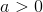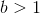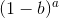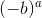## Example Questions

### Example Question #1 : How To Subtract Exponents

Simplify: 32 * (423 - 421)

3^3 * 4^21

3^21

4^4

3^3 * 4^21 * 5

3^3 * 4^21 * 5

Explanation:

Begin by noting that the group (423 - 421) has a common factor, namely 421.  You can treat this like any other constant or variable and factor it out.  That would give you: 421(42 - 1). Therefore, we know that:

32 * (423 - 421) = 32 * 421(42 - 1)

Now, 42 - 1 = 16 - 1 = 15 = 5 * 3.  Replace that in the original:

32 * 421(42 - 1) = 32 * 421(3 * 5)

Combining multiples withe same base, you get:

33 * 421 * 5

### Example Question #51 : Exponents

Quantitative Comparison

Quantity A: 64 – 32

Quantity B: 52 – 42

Quantity B is greater.

The relationship cannot be determined from the information given.

The two quantities are equal.

Quantity A is greater.

Quantity A is greater.

Explanation:

We can solve this without actually doing the math. Let's look at 64 vs 52. 64 is clearly bigger. Now let's look at 32 vs 42. 32 is clearly smaller. Then, bigger – smaller is greater than smaller – bigger, so Quantity A is bigger.

### Example Question #1561 : Gre Quantitative Reasoning, andis odd.Quantity A:Quantity B:The two quantities are equal.

The relationship cannot be determined from the information given.

Quantity B is greater.

Quantity A is greater.

Quantity A is greater.

Explanation:

The first thing to note is the relationship between (–b) and (1 – b):

(–b) < (1 – b) because (–b) + 1 = (1 – b).

Now when b > 1, (1 – b) < 0 and –b < 0. Therefore (–b) < (1 – b) < 0.

Raising a negative number to an odd power produces another negative number.

Thus (–b)a < (1 – b)a < 0.